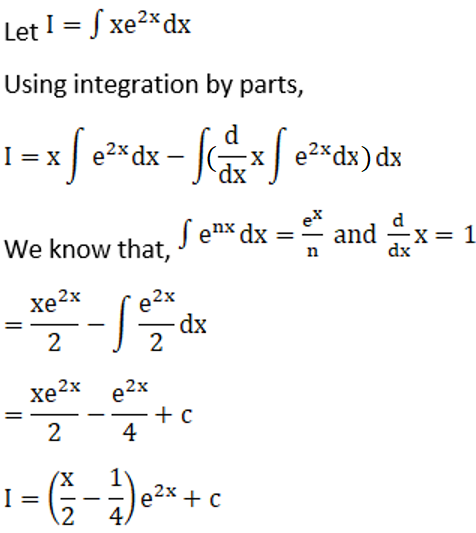# RD Sharma Solutions For Class 12 Maths Exercise 19.25 Chapter 19 Indefinite Integrals

RD Sharma Solutions for Class 12 Maths Exercise 19.25 Chapter 19 Indefinite Integrals is provided here. In this exercise, we shall discuss the topic of integration of parts by using ILATE rule with a huge number of problems. Solutions for such problems are derived in a step by step format by our experts to help students understand the concepts and come out with good results in their examinations.

RD Sharma Maths Solutions for Class 12 prepared by our experts is the best reference module one can use to secure good marks in their exams. Students can refer and easily download the pdf RD Sharma Solutions for Class 12 Maths Chapter 19 Indefinite Integrals Exercise 19.25 for free, from the links given below.

## Download the PDF of RD Sharma Solutions For Class 12 Chapter 19 – Indefinite Integrals Exercise 19.25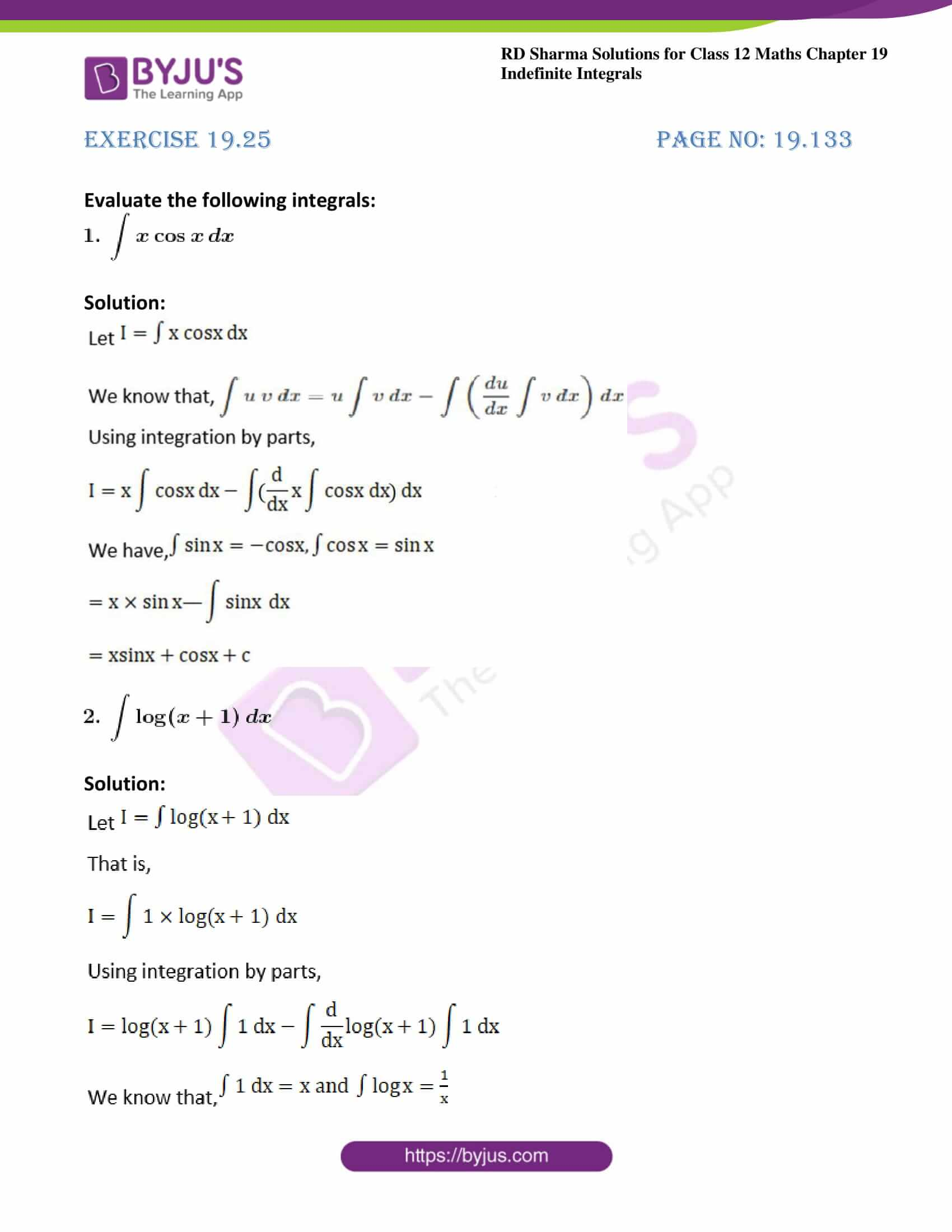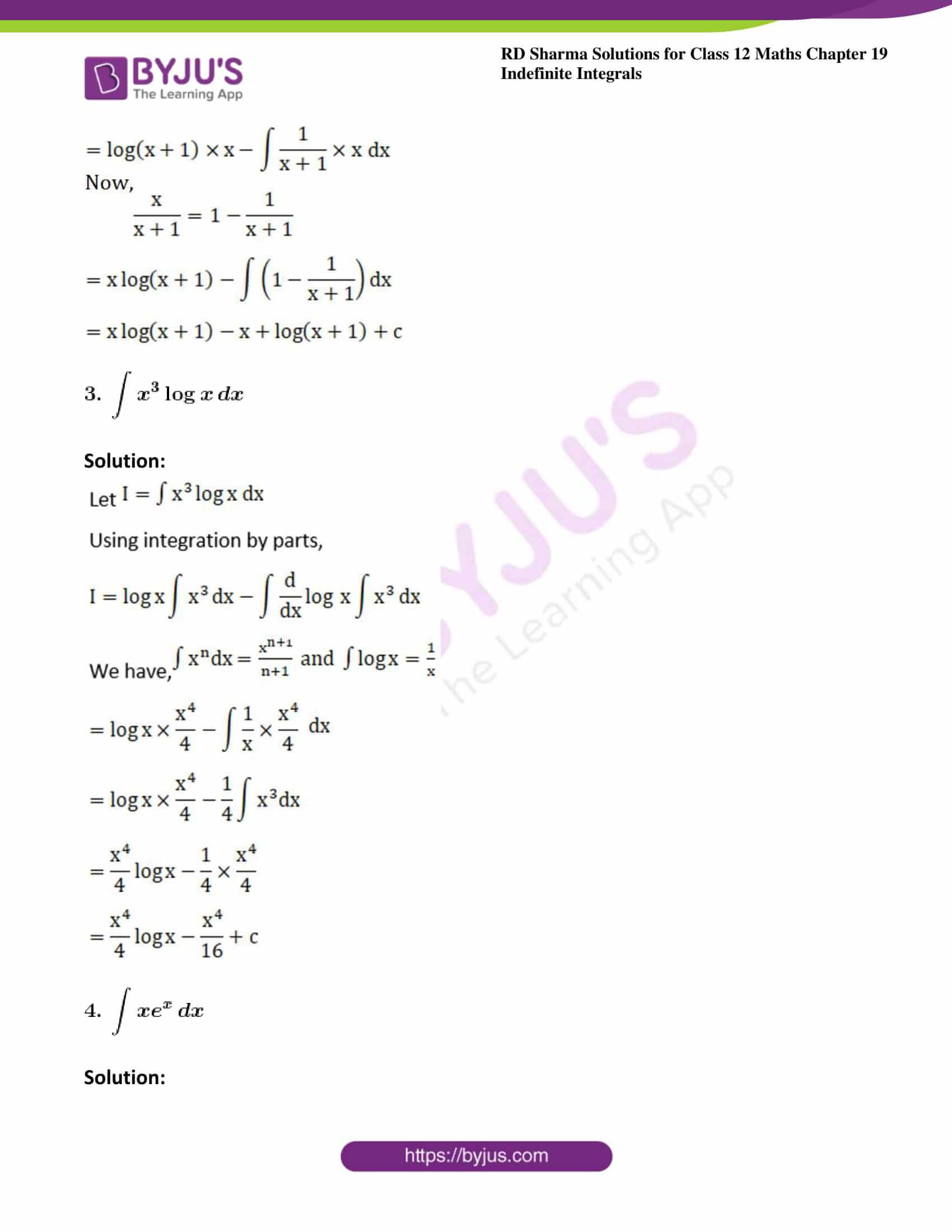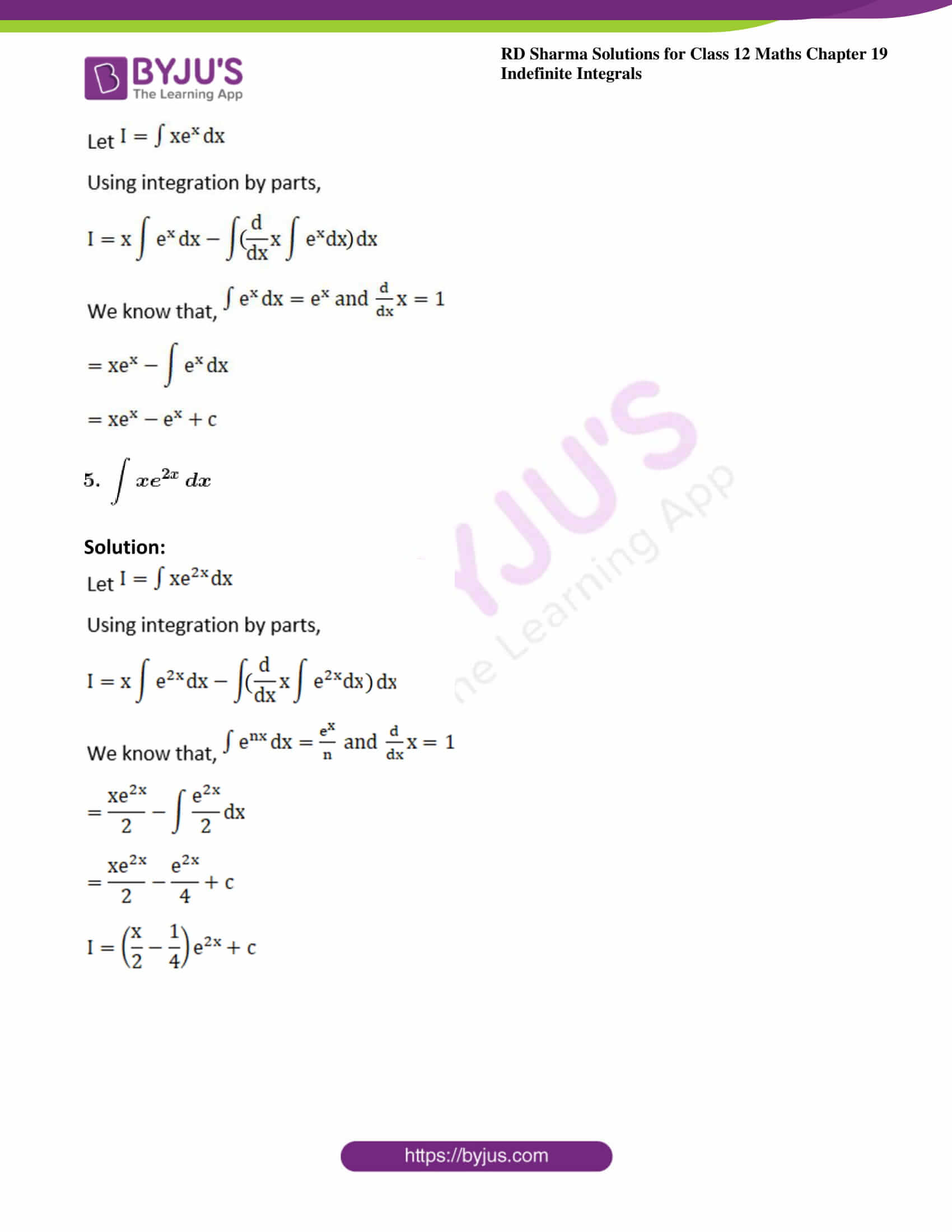### Access other exercises of RD Sharma Solutions For Class 12 Chapter 19 – Indefinite Integrals

Exercise 19.1 Solutions

Exercise 19.2 Solutions

Exercise 19.3 Solutions

Exercise 19.4 Solutions

Exercise 19.5 Solutions

Exercise 19.6 Solutions

Exercise 19.7 Solutions

Exercise 19.8 Solutions

Exercise 19.9 Solutions

Exercise 19.10 Solutions

Exercise 19.11 Solutions

Exercise 19.12 Solutions

Exercise 19.13 Solutions

Exercise 19.14 Solutions

Exercise 19.15 Solutions

Exercise 19.16 Solutions

Exercise 19.17 Solutions

Exercise 19.18 Solutions

Exercise 19.19 Solutions

Exercise 19.20 Solutions

Exercise 19.21 Solutions

Exercise 19.22 Solutions

Exercise 19.23 Solutions

Exercise 19.24 Solutions

Exercise 19.26 Solutions

Exercise 19.27 Solutions

Exercise 19.28 Solutions

Exercise 19.29 Solutions

Exercise 19.30 Solutions

Exercise 19.31 Solutions

Exercise 19.32 Solutions

### Exercise 19.25 Page No: 19.133

Evaluate the following integrals: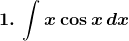Solution: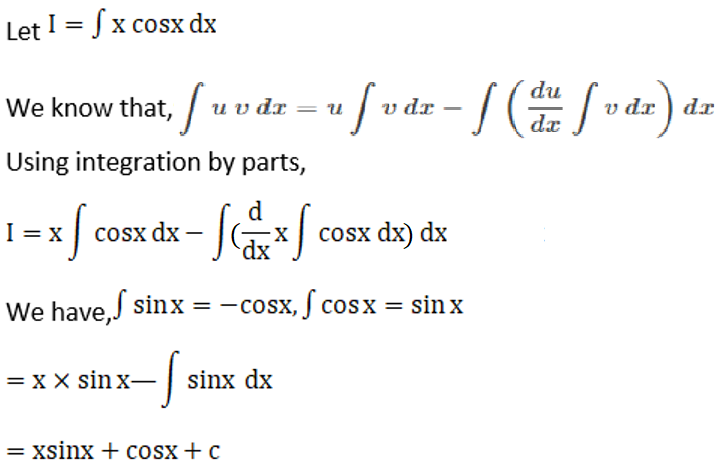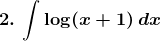Solution: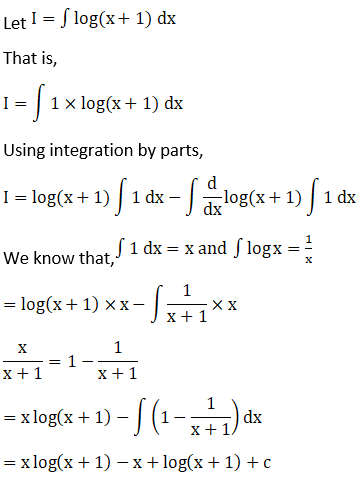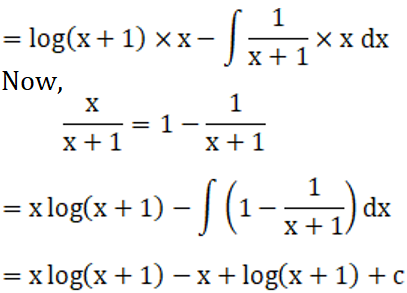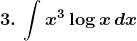Solution: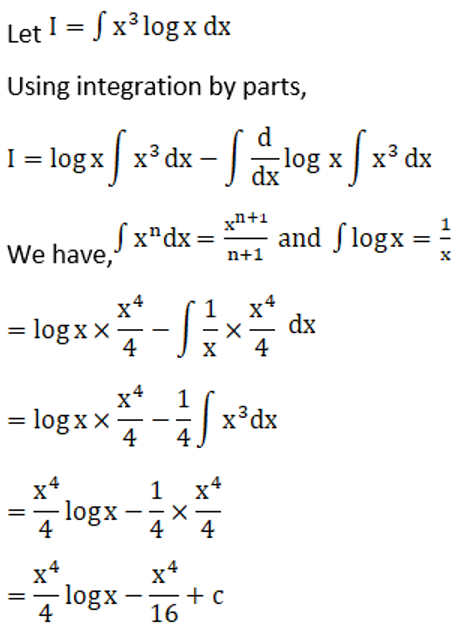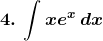Solution: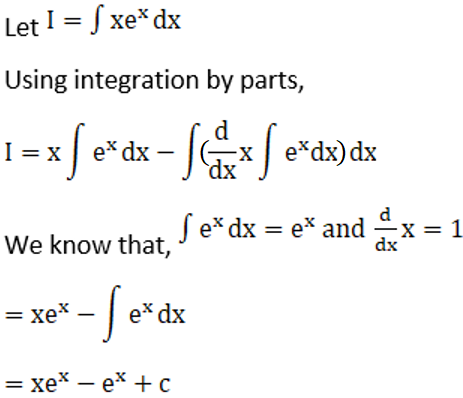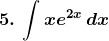Solution: